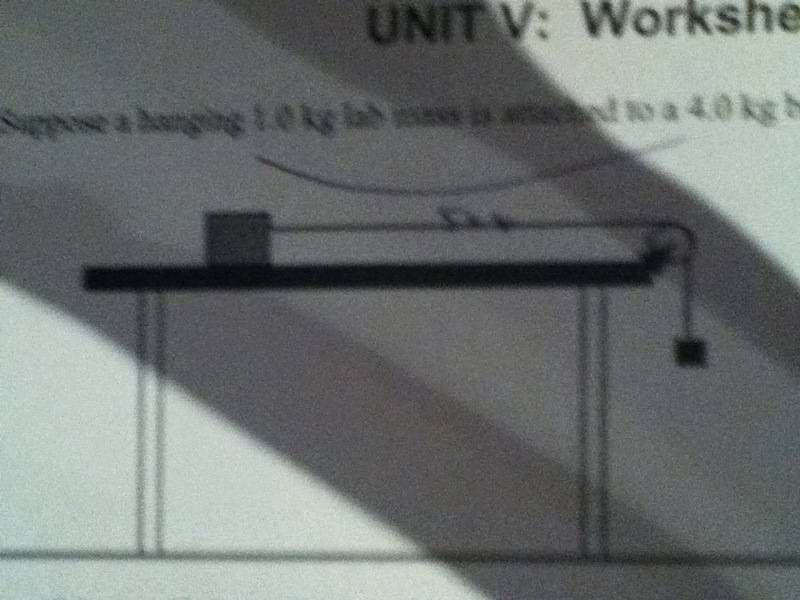# Finding the mininum value of the coffecitent of friction

jtwitty

## Homework Statement

1. Suppose a hanging 1.0kg lab mass is attached to a 4.0kg block on the table

(The picture)a. If the coefficient of kinetic friction, $$\mu$$k is .2, what is the acceleration?

b. What would the mininum value of the coefficient of static friction, $$\mu$$s, in order for the block to remian motionless

## Homework Equations

a = f/m
$$\mu$$ = frictional force / normal force

## The Attempt at a Solution

a) .2 = friction/50 (i got 50 from 5 [the two blocks] multiplied by gravity)... friction = 10
a = 10/5
a = 2m/s

b) idk how to figure this out?

MasterMatt
You need to look at both blocks as a system.

Friction Force due to gravity on the hanging block.
<------------------(mass of both blocks)----------------------------------------------->

Keep in mind that a=f/m isn't really an equation. F=ma is the sum of all forces on an object or system. With that being said you can now use that equation as follows:

F=ma
Fg-Ff=ma....and I'm sure you can finish the rest.

jtwitty
i meant

a = $$\Sigma$$f / m

thats a real equation